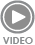# What’s the difference between moment and torque in linear motion applications?

Torque produces rotation about an axis or pivot point.

In linear motion, we often deal with applications that involve forces applied at a distance from a linear guide — referred to as cantilevered, or moment, loads. In these cases, we’re concerned with guide’s moment load capacity, or its ability to resist rotating. But we also deal with components that should rotate when a force is applied at a distance, such as a ball screw shaft transmitting torque from a motor to drive a load. In these cases, we’re concerned with the amount of torque the component is able to transmit.

Both the moment on the linear guide and the torque on the shaft are caused by forces applied at a distance, and both are measured in units of Newton-meters (Nm) or pound-feet (lb-ft). So what’s the difference between the moment applied to the linear guide and the torque applied to the screw shaft?

The primary difference between moment and torque can be found by studying the object’s reaction. When torque is applied to a shaft, the shaft rotates. But when a moment load is applied to a linear guide, the guide remains stationary (unless the moment exceeds the guide’s rated moment capacity, in which case, the guide may deform or begin to rotate).

In other words, torque causes a change in the object’s angular momentum, which produces rotation. A moment, on the other hand, does not produce a change in angular momentum. The body to which the moment is applied remains stationary, and reaction forces that arise within the object and its supporting members prevent the object from rotating.

For example, a load applied to an end-supported cantilever beam will cause a reaction force and a bending moment on the beam, but doesn’t change its angular momentum, and and therefore, doesn’t cause the beam to rotate.

Because moment forces are static — they don’t result in motion — they can be resolved into reaction forces that counteract the applied moment.

The amount of torque applied to a shaft is is found by multiplying the applied force by the moment arm, which is the perpendicular distance between the pivot point (or axis of rotation) and the force.

If the applied force isn’t perpendicular to the pivot point or axis of rotation, the angle of the force must be taken into account to find the length of the moment arm.xyz linear pick and place motion system